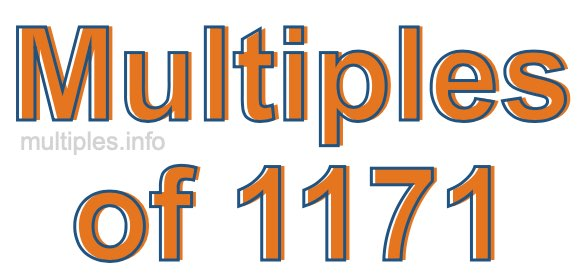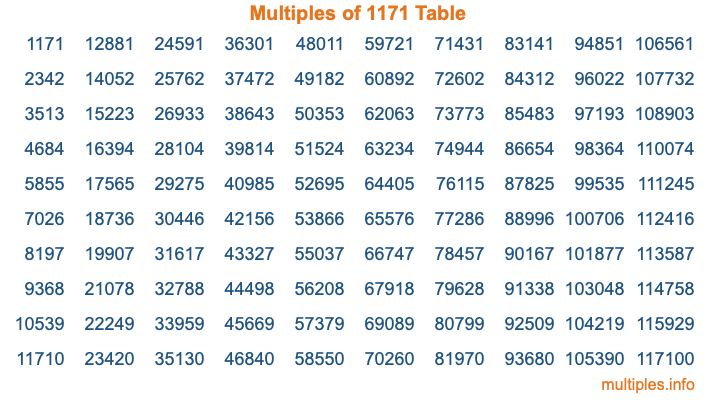Multiples of 1171Welcome to the Multiples of 1171 page. Here we will first teach you everything you will ever need to know about the multiples of 1171, and then give you a study guide summary of everything we taught you to make sure you remember it all. Use this page to look up facts and learn information about the multiples of 1171. This page will make you a multiples of one thousand one hundred seventy-one expert!

Definition of Multiples of 1171
Multiples of 1171 are all the numbers that when divided by 1171 equal an integer. Each of the multiples of 1171 are called a multiple. A multiple of 1171 is created by multiplying 1171 by an integer.

Therefore, to create a list of multiples of 1171, you start with 1 multiplied by 1171, then 2 multiplied by 1171, then 3 multiplied by 1171, and so on for as long as you want. Thus, the list of the first five multiples of 1171 is 1171, 2342, 3513, 4684, and 5855. To see a larger list of multiples of 1171, see the printable image of Multiples of 1171 further down on this page. We also have a category where you can choose any nth multiple of 1171.

Multiples of 1171 Checker
The Multiples of 1171 Checker below checks to see if any number of your choice is a multiple of 1171. In other words, it checks to see if there is any number (integer) that when multiplied by 1171 will equal your number. To do that, we divide your number by 1171. If the the quotient is an integer, then your number is a multiple of 1171.

Is  a multiple of 1171?

Least Common Multiple of 1171 and ...
A Least Common Multiple (LCM) is the lowest multiple that two or more numbers have in common. This is also called the smallest common multiple or lowest common multiple and is useful to know when you are adding our subtracting fractions. Enter one or more numbers below (1171 is already entered) to find the LCM.

Check out our LCM Calculator if you need more details about the Least Common Multiple or if you need the LCM for different numbers for adding and subtraction fractions.

nth Multiple of 1171
As we stated above, 1171 is the first multiple of 1171, 2342 is the second multiple of 1171, 3513 is the third multiple of 1171, and so on. Enter a number below to find the nth multiple of 1171.

th multiple of 1171

Multiples of 1171 vs Factors of 1171
1171 is a multiple of 1171 and a factor of 1171, but that is where the similarities end. All postive multiples of 1171 are 1171 or greater than 1171. All positive factors of 1171 are 1171 or less than 1171.

Below is the beginning list of multiples of 1171 and the factors of 1171 so you can compare:

Multiples of 1171: 1171, 2342, 3513, 4684, 5855, etc.

Factors of 1171: 1, 1171

As you can see, the multiples of 1171 are all the numbers that you can divide by 1171 to get a whole number. The factors of 1171, on the other hand, are all the whole numbers that you can multiply by another whole number to get 1171.

It's also interesting to note that if a number (x) is a factor of 1171, then 1171 will also be a multiple of that number (x).

Multiples of 1171 vs Divisors of 1171
The divisors of 1171 are all the integers that 1171 can be divided by evenly. Below is a list of the divisors of 1171.

Divisors of 1171: 1, 1171

The interesting thing to note here is that if you take any multiple of 1171 and divide it by a divisor of 1171, you will see that the quotient is an integer.

Multiples of 1171 Table
Below is an image of the first 100 multiples of 1171 in a table. The table is in chronological order, column by column. The first column has the first ten multiples of 1171, the second column has the next ten multiples of 1171, and so on.The Multiples of 1171 Table is also referred to as the 1171 Times Table or Times Table of 1171. You are welcome to print out our table for your studies.

Negative Multiples of 1171
Although not often discussed or needed in math, it is worth mentioning that you can make a list of negative multiples of 1171 by multiplying 1171 by -1, then by -2, then by -3, and so on, to get the following list of negative multiples of 1171:

-1171, -2342, -3513, -4684, -5855, etc.

Multiples of 1171 Summary
Below is a summary of important Multiples of 1171 facts that we have discussed on this page. To retain the knowledge on this page, we recommend that you read through the summary and explain to yourself or a study partner why they hold true.

There are an infinite number of multiples of 1171.

A multiple of 1171 divided by 1171 will equal a whole number.

1171 divided by a factor of 1171 equals a divisor of 1171.

The nth multiple of 1171 is n times 1171.

The largest factor of 1171 is equal to the first positive multiple of 1171.

1171 is a multiple of every factor of 1171.

1171 is a multiple of 1171.

A multiple of 1171 divided by a divisor of 1171 equals an integer.

1171 divided by a divisor of 1171 equals a factor of 1171.

Any integer times 1171 will equal a multiple of 1171.

Multiples of a Number
Here you can get the multiples of another number, all with the same attention to detail as we did for multiples of 1171 on this page.

Multiples of
Multiples of 1172
Did you find our page about multiples of one thousand one hundred seventy-one educational? Do you want more knowledge? Check out the multiples of the next number on our list!